Solving Wordle with Z3

I've been enjoying this viral new word game eponymously named Wordle. The aim of the game is to guess the day's word in six or less tries. I'll go through an example game to give an idea of the rules.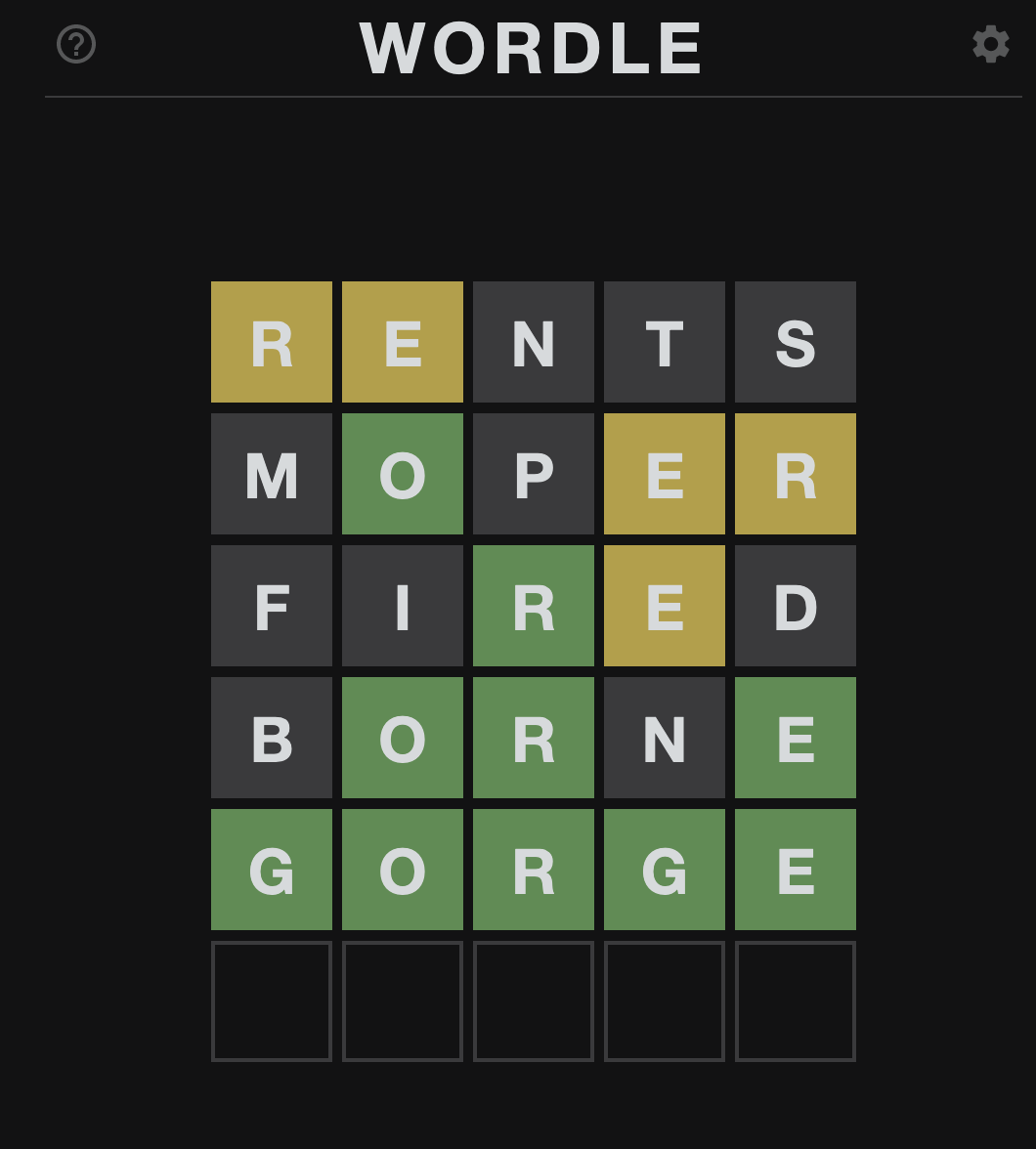In this particular game:

• the hidden word was G O R G E, guessed correctly on the fifth try.
• the first guess was R E N T S, which was incorrect. However it gave us clues: R is found withing in the correct word, but not at this location (same with E) and N, T, S are not found in the correct word.
• the second guess confirms that O appears in the correct word and at the same location we guessed.
• Note that letters are allowed to appear more than once in the correct word - however if a letter appears only once, but our guess contains that letter multiple times, all except one instance will be marked gray.
• Armed with these rules and an English dictionary, we can encode these rules as constraint satisfaction program and try to solve it using an SMT solver. My go to SMT solver is Z3 because it is simple to install, has well-made python bindings and an easy to use API.

With pip, cmake and a C++ compiler as prerequisites, you can simply install z3 with the following command:

\$ pip install z3-solver

My goal is to model this problem with variables and constraints that are easy to understand - hopefully helpful for people who are new to SMT solvers. Let's first start by instantiating the z3 solver class and constructing our variables:

import z3

word_length = 5

def define_letter_variables():
return [z3.Int(f"letter_{index}") for index in range(word_length)]

if __name__ == "__main__":
solver = z3.Solver()
letter_vars = define_letter_variables()

We are going to model each letter of our guess as a separate z3.Int. z3 can represent arbitrarily large integers - but we only need to model each variable as a letter - ranging from 0 - 25 for the English alphabet. To model our variables in that legal range, let's add some constraints:
for letter_var in letter_vars:
solver.add(letter_var >= 0, letter_var <= 25)

return solver

Just to sanity check our work so far, let's run the solver and see what it gives us:
letter_to_index_map = {letter: index for index, letter in enumerate("abcdefghijklmnopqrstuvwxyz")}
index_to_letter_map = {index: letter for letter, index in letter_to_index_map.items()}

def pretty_print_solution(model, letter_vars):
word = []
for index, letter_var in enumerate(letter_vars):
word.append(index_to_letter_map[model[letter_var].as_long()])

print(''.join(word))

if __name__ == "__main__":
solver = z3.Solver()
letter_vars = define_letter_variables()

print("Solving...")
result = solver.check()
print(result)
assert result == z3.sat

model = solver.model()
pretty_print_solution(model, letter_vars)

\$ python wordle.py
Solving...
sat
aaaaa

A pretty boring result. The solver decided to set each of our letter variables to 0, resulting in the final word guess of aaaaa.

We'll need to teach the solver about English if we want it to pick words that actually make sense. We can use the dictionary that UNIX-like OS's come with:

def remove_plurals(words):
five_letter_words = list(filter(lambda word: len(word) == 5, words))
four_letter_words = set(filter(lambda word: len(word) == 4, words))
all_five_letter_words_ending_in_s = set(filter(lambda word: word == "s", five_letter_words))
singular_five_letter_words = list(filter(lambda word: not (word in all_five_letter_words_ending_in_s and word[:4] in four_letter_words), five_letter_words))
return singular_five_letter_words

def remove_words_with_invalid_chars(words):
valid_chars_set = set(letter_to_index_map.keys())

def contains_only_valid_chars(word):
return set(word).issubset(valid_chars_set)

return filter(contains_only_valid_chars, words)

with open(dictionary_path, "r") as f:
all_legal_words = set(word.strip() for word in f.readlines())

words = remove_words_with_invalid_chars(all_legal_words)
words = list(words)
words = remove_plurals(words)
return words

if __name__ == "__main__":
...

Now that we have loaded the dictionary, cleaned up invalid characters and removed plurals (I've found that Wordle only uses singular words for answer words, if you found otherwise let me know!), we can add constraints to force the solver to pick only legal words.
all_words_disjunction = []

for word in words:
word_conjuction = z3.And([letter_vars[index] == letter_to_index_map[letter] for index, letter in enumerate(word)])
all_words_disjunction.append(word_conjuction)

return solver

if __name__ == "__main__":
...

The add_legal_words_constraints function seems innocuous, but it adds a whole bunch of constraints and makes up the meat of the solver. Read in boolean logic, you can think of the solver now having the following constraints, given our dictionary only consisted of two words: "hello" and "world".

(letter_0 == "h" and letter_1 == "e" and letter_2 == "l" and letter_3 == "l" and letter_4 == "o") or
(letter_0 == "w" and letter_1 == "o" and letter_2 == "r" and letter_3 == "l" and letter_4 == "d")

Now we are ready to run the solver and get valid guesses only! Let's give it a try:
\$ python wordle.py
Solving...
sat

Awesome! We get badge as our guess. We can input that into Wordle and see what we get. For the Wordle on January 10th, 2022, I get the following result: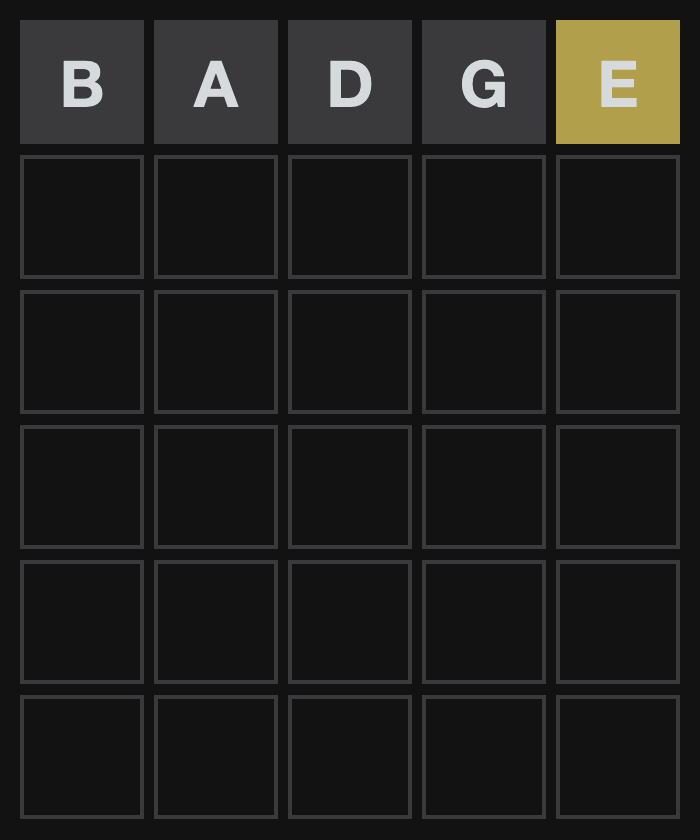A relatively bad hit - we got 4 letters wrong and only one right, but in the wrong position. Let's add constraints to the solver to prevent it from picking these letters again and tell it that it should definitely consider E in it's future guesses.
for letter_var in letter_vars:

return solver

solver.add(z3.Or([letter_var == letter_to_index_map[letter] for letter_var in letter_vars]))

return solver

return solver

if __name__ == "__main__":
...

solver = add_invalid_position_constraint(solver, letter_vars, "e", 4)

We prevent B A D G from appearing in any position and tell the solver that while E must appear in one of the positions, it can't appear in the fifth position. Let's run the solver again:
\$ python wordle.py
Solving...
sat
every

Okay, great. It kept the E, but not at the fifth position and didn't guess either of B A D G. Let's input that guess and see what we get.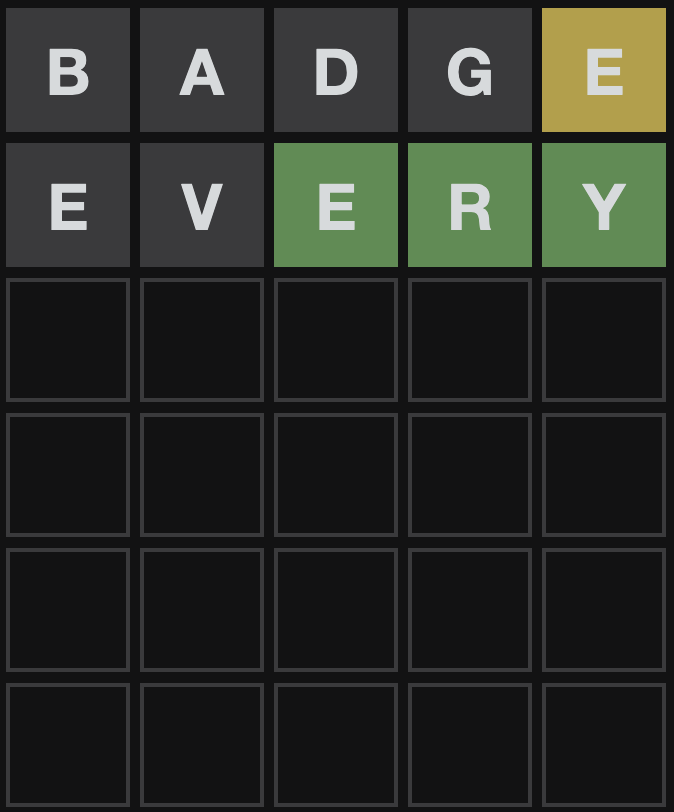Wonderful, we got an exact match for E R Y. Note that the E in the first position is grayed out - this means that it should appear only once in this word. Let's add a constraint for that as well as adding more banning constraints.

return solver

unique_letter_disjunction = []

for letter_var in letter_vars:
this_letter_conjunction = [letter_var == letter_to_index_map[letter]]
for other_letter_var in letter_vars:
if letter_var == other_letter_var:
continue
this_letter_conjunction.append(other_letter_var != letter_to_index_map[letter])
unique_letter_disjunction.append(z3.And(this_letter_conjunction))

return solver

if __name__ == "__main__":
...

solver = add_exact_letter_position_constraint(solver, letter_vars, "e", 2)
solver = add_exact_letter_position_constraint(solver, letter_vars, "r", 3)
solver = add_exact_letter_position_constraint(solver, letter_vars, "y", 4)

With these new constraints, running the solver produces the guess:
\$ python wordle.py
Solving...
sat
fiery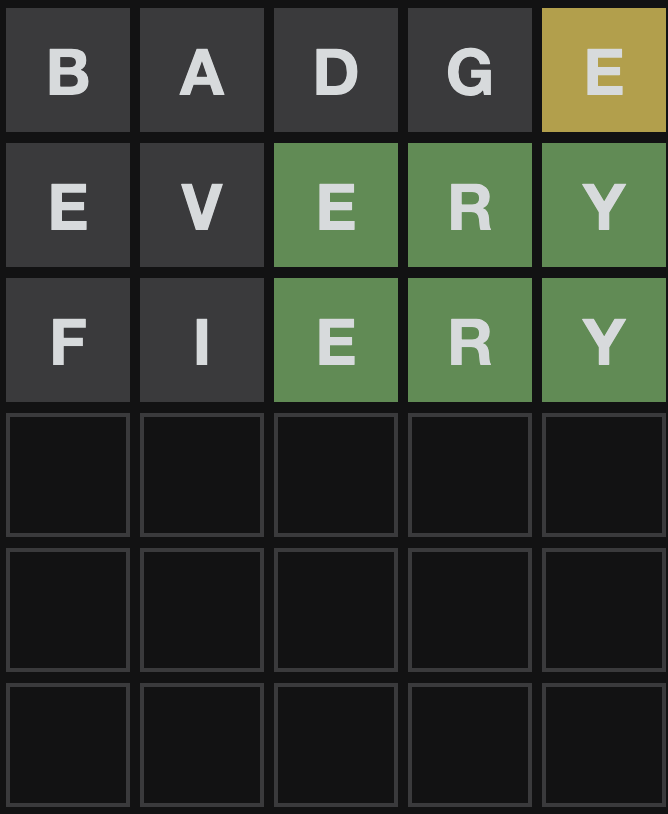A complete miss :(. Let's prevent the solver from guessing both these letters:

if __name__ == "__main__":
...
...

\$ python wordle.py
Solving...
sat
query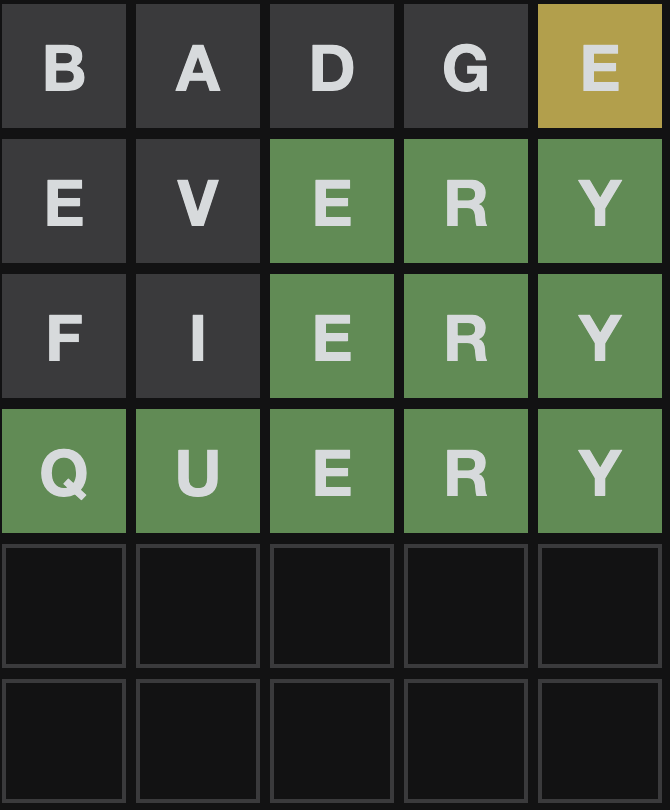Yay! The solver guessed the correct word: Q U E R Y

While the modeling we have so far works pretty well, we can further improve it by taking into account letter frequencies (how often a letter appears across words) and word frequencies (how often a word appears in a large corpus). In my testing I also found that the solver tended to guess words that I wouldn't really go for because they're words that, while legal, are obscure and never really used in common English. Wordle tends to use words that are not very obscure, but also not very common. We can use z3's optimization features to encode a cost function - valuing guesses that contain more frequent letters early on and more obscure words later as we have more information about the word's makeup.

Here's a snippet of such a model:

def optimize_search(solver, word_to_freq, letter_vars):

def maximize_letter_frequency():
normalized_letter_frequency_map = make_normalized_letter_frequency_map(letter_frequency_map)

for freq_var, letter_var in zip(letter_frequency_vars, letter_vars):
for letter_index, letter in index_to_letter_map.items():
solver.add(z3.Implies(letter_var == letter_index, freq_var == normalized_letter_frequency_map[letter]))

def maximize_word_frequency():
normalized_word_frequency_map = make_normalized_word_frequency_map(word_to_freq)

for word, freq in normalized_word_frequency_map.items():
word_conjuction = z3.And([letter_vars[index] == letter_to_index_map[letter] for index, letter in enumerate(word)])

letter_frequency_vars = [z3.Real(f"letter_{index}_frequency") for index in range(len(letter_vars))]
letter_frequency_sum = z3.Real(f"letter_frequency_sum")

word_frequency = z3.Real(f"word_frequency")

# Each letter frequency can be [0, 1.0], we divide the sum by num of letters to get a normalized sum